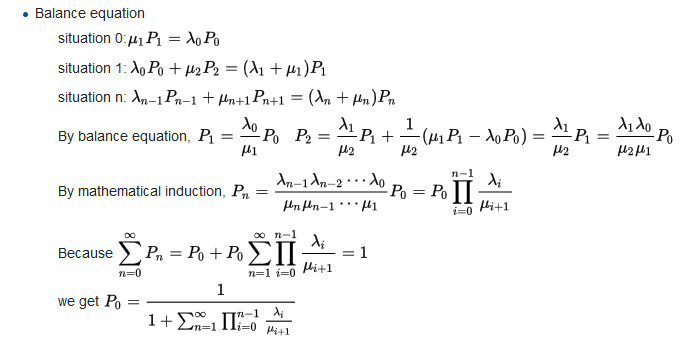# How Simulation Software is Making Queueing Theory Irrelevant

Date: 8/30/2016
Author: Descreye Solutions

Queueing theory has been used for over 100 years to analyze system dynamics. Queueing theory uses a series of mathematical equations to estimate wait time in queues. It has been an important part of system analysis because it simplifies complex systems into a series of equations. However, the problem remains that with increased system complexity, queueing theory becomes inadequate for estimating the effects of changes on the system. Below is the proof for the equation to determine the probability that 0 objects are in a M/M/1 queue given n people in the system. Remember that this is an extremely simple problem....Due to the unrealistic nature of trying to boil entire systems down into one equation, queueing theory has always been unable to solve the difficult system dynamic problems. In queueing theory, when the system contained many interrelated processes it became nearly impossible to perform the analysis.

However, just because queueing theory isn't capable does not mean all is lost. Over the years there has been a general transition from queueing theory to simulation, because simulation is capable of doing the analysis that is necessary for complex systems. Unlike queueing theory, simulation does not attempt to mathematically solve the system. Instead simulation models are built to emulate the system. The models are then run across multiple replications in order to observe the resultant variability. Additionally simulation has the ability to observe many factors like resource utilization, total system time, and WIP that were nearly impossible to estimate using pure queueing theory.

While queueing theory is still an interesting mathematical problem it no longer seems to be a useful tool for those involved in process improvement, because simulation is a more capable tool for analyzing the complexities involved in real-world systems.

© Descreye Solutions, 2022